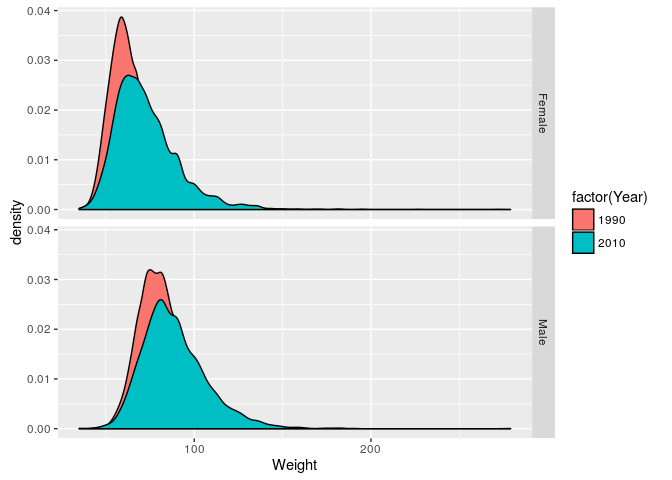# 1 Colors

Colors should reflect the nature of the data and be carefully chosen to convey equivalent information to all viewers. The RColorBrewer package provides an easy way to choose colors; see also the colorbrewer2 web site.

library(RColorBrewer)
display.brewer.all()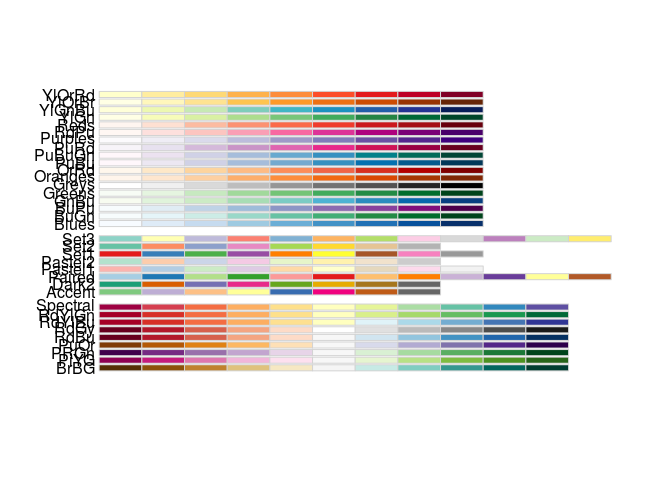We’ll use a color scheme from the ‘qualitative’ series, to represent different levels of factors and for choice of colors. We’ll get the first four colors.

palette <- brewer.pal(4, "Dark2")

# 2 ‘Base’ Graphics

We’ll illustrate ‘base’ graphics using the built-in mtcars data set

data(mtcars)     # load the data set
head(mtcars)
##                    mpg cyl disp  hp drat    wt  qsec vs am gear carb
## Mazda RX4         21.0   6  160 110 3.90 2.620 16.46  0  1    4    4
## Mazda RX4 Wag     21.0   6  160 110 3.90 2.875 17.02  0  1    4    4
## Datsun 710        22.8   4  108  93 3.85 2.320 18.61  1  1    4    1
## Hornet 4 Drive    21.4   6  258 110 3.08 3.215 19.44  1  0    3    1
## Hornet Sportabout 18.7   8  360 175 3.15 3.440 17.02  0  0    3    2
## Valiant           18.1   6  225 105 2.76 3.460 20.22  1  0    3    1

The basic model is to plot data, e.g., the relationshiop between miles per gallon and horsepower.

plot(mpg ~ hp, mtcars)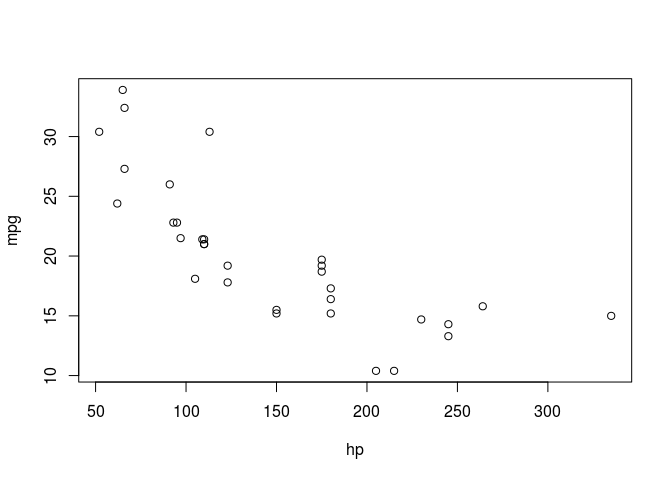The appearance can be influenced by arguments, see ?plot then ?plot.default and par.

plot(mpg ~ hp, mtcars, pch=20, cex=2, col=palette)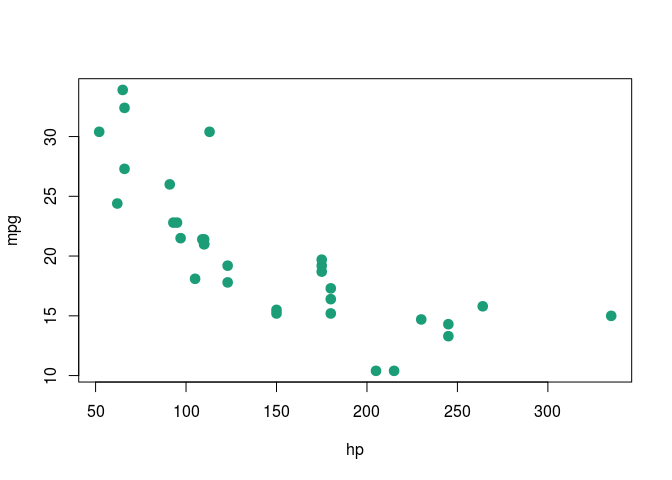More complicated plots can be composed via a series of commands, e.g., to plot a linear regression, make the plot, and add the regression line using abline().

plot(mpg ~ hp, mtcars)
fit <- lm(mpg ~ hp, mtcars)
abline(fit, col=palette, lwd=3)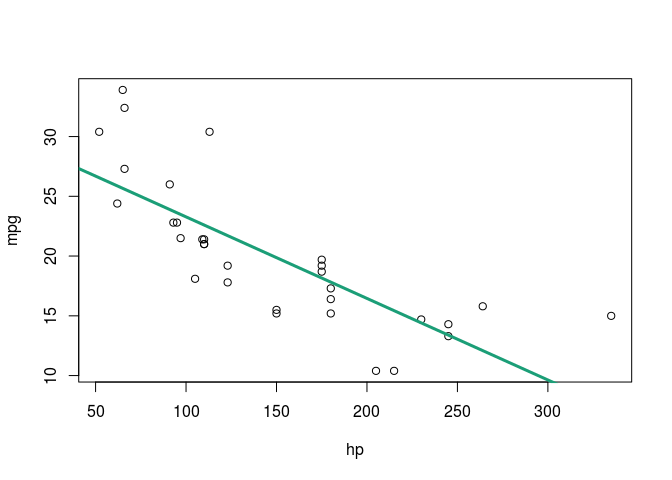# 3ggplot2 Graphics

library(ggplot2)

## 3.1 Basics

Tell ggplot2 what to plot using ggplot() and aes(); we’ll use the columns hp (horsepower) and mpg (miles per gallon).

ggplot(mtcars, aes(x=hp, y=mpg))

Note the neutral gray background with white gridlines to provide unobtrusive orientation. Note the relatively small size of the axis and tick labels, to avoid distracting from the pattern provided by the data.

ggplot2 uses different geom_* to add to the basic plot. Add points

ggplot(mtcars, aes(x=hp, y=mpg)) + geom_point()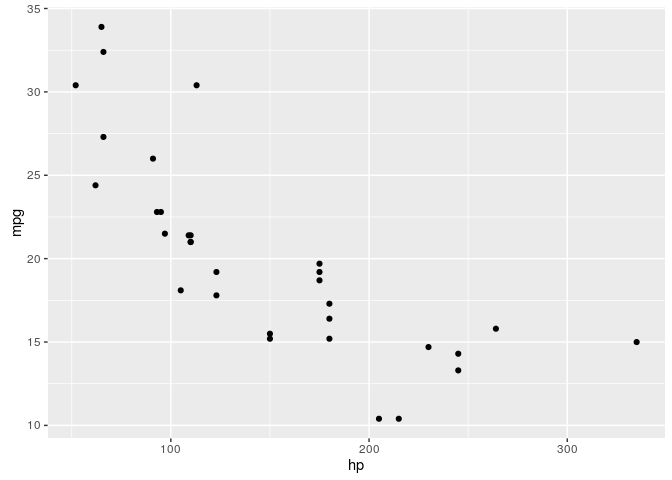Add a linear regression line and standard error…

ggplot(mtcars, aes(x=hp, y=mpg)) + geom_point() +
geom_smooth(method=lm, col=palette)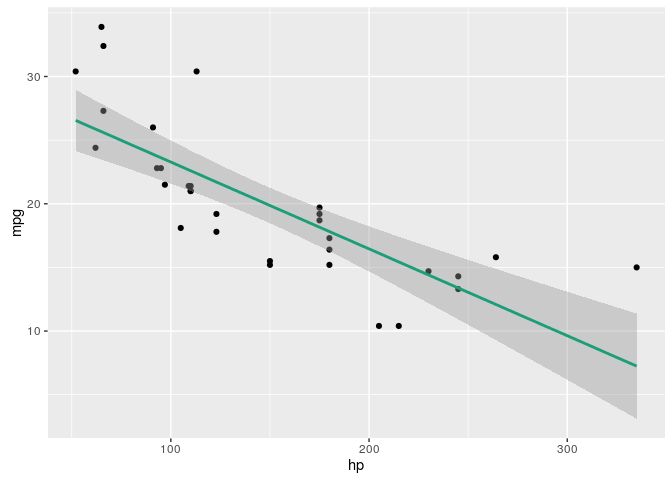…and a locally smoothed regression

ggplot(mtcars, aes(x=hp, y=mpg)) + geom_point() +
geom_smooth(method=lm, col=palette) +
geom_smooth(col=palette)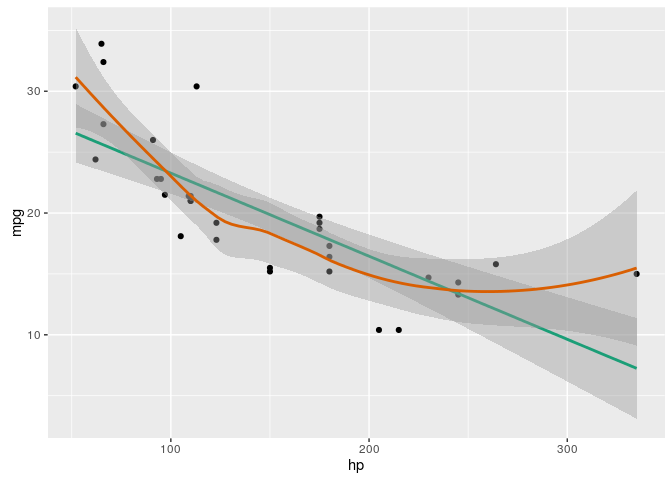## 3.2 Density plots

path <- file.choose()
brfss <- read.csv(path)

Plot the distribution of weights using geom_density()

ggplot(brfss, aes(x=Weight)) + geom_density()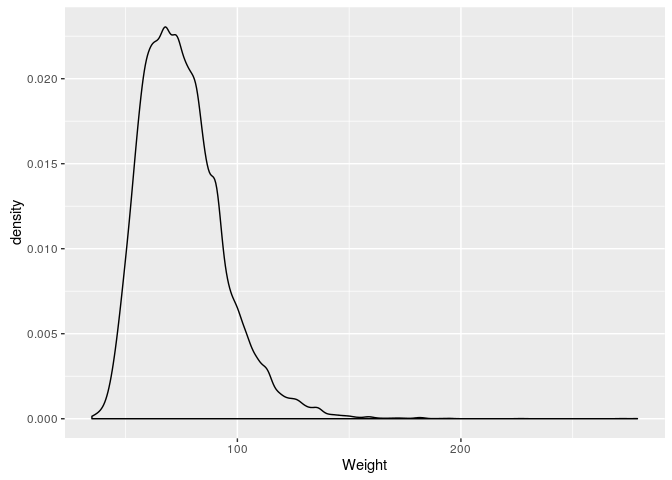Plot the weights separately for each year, using fill=factor(Year) and alpha=.5 arguments in the aes() argument

ggplot(brfss, aes(x=Weight, fill=factor(Year))) +
geom_density(alpha=0.5)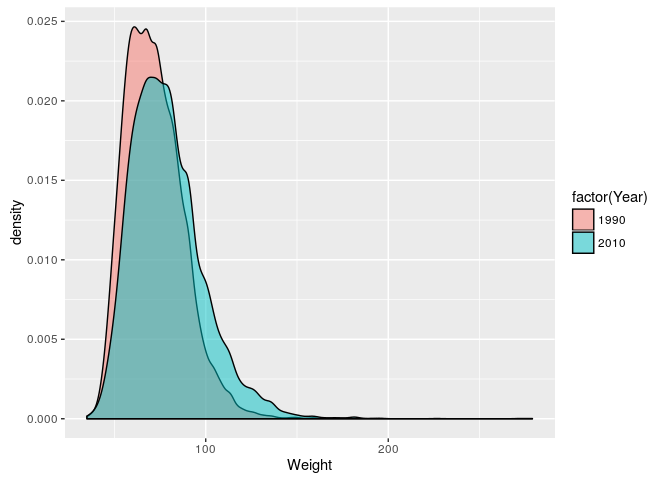Americans are getting heavier, and the variation in weights is increasing.

## 3.3 Facets

Create separate panels for each sex using facet_grid(), with a formula describing the factor(s) to use for rows (left-hand side of the formula) and columns (right-hand side).

ggplot(brfss, aes(x=Weight, fill=factor(Year))) +
geom_density() +
facet_grid(Sex ~ .)